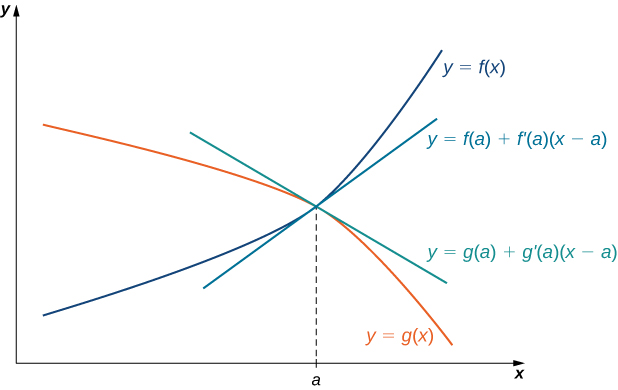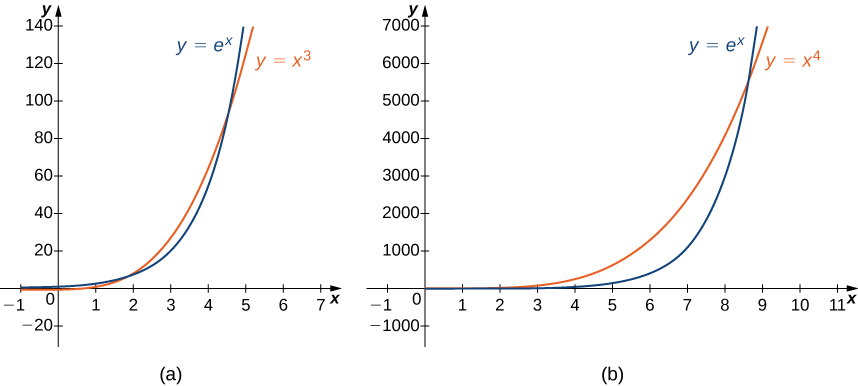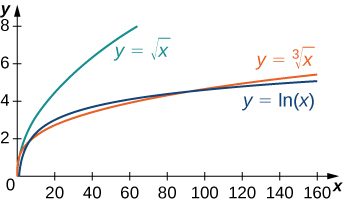## 4.5 L’Hôpital’s Rule

### Learning Objectives

• Recognize when to apply L’Hôpital’s rule.
• Identify indeterminate forms produced by quotients, products, subtractions, and powers, and apply L’Hôpital’s rule in each case.
• Describe the relative growth rates of functions.

In this section, we examine a powerful tool for evaluating limits. This tool, known as L’Hôpital’s rule, uses derivatives to calculate limits. With this rule, we will be able to evaluate many limits we have not yet been able to determine. Instead of relying on numerical evidence to conjecture that a limit exists, we will be able to show definitively that a limit exists and to determine its exact value.

# Applying L’Hôpital’s Rule

L’Hôpital’s rule can be used to evaluate limits involving the quotient of two functions. Consider

$\underset{x\to a}{\lim}\frac{f(x)}{g(x)}$.

If $\underset{x\to a}{\lim}f(x)=L_1$ and $\underset{x\to a}{\lim}g(x)=L_2 \ne 0$, then

$\underset{x\to a}{\lim}\frac{f(x)}{g(x)}=\frac{L_1}{L_2}$.

However, what happens if $\underset{x\to a}{\lim}f(x)=0$ and $\underset{x\to a}{\lim}g(x)=0$? We call this one of the indeterminate forms, of type $\frac{0}{0}$. This is considered an indeterminate form because we cannot determine the exact behavior of $\frac{f(x)}{g(x)}$ as $x\to a$ without further analysis. We have seen examples of this earlier in the text. For example, consider

$\underset{x\to 2}{\lim}\frac{x^2-4}{x-2}$ and $\underset{x\to 0}{\lim}\frac{ \sin x}{x}$.

For the first of these examples, we can evaluate the limit by factoring the numerator and writing

$\underset{x\to 2}{\lim}\frac{x^2-4}{x-2}=\underset{x\to 2}{\lim}\frac{(x+2)(x-2)}{x-2}=\underset{x\to 2}{\lim}(x+2)=2+2=4$.

For $\underset{x\to 0}{\lim}\frac{\sin x}{x}$ we were able to show, using a geometric argument, that

$\underset{x\to 0}{\lim}\frac{\sin x}{x}=1$.

Here we use a different technique for evaluating limits such as these. Not only does this technique provide an easier way to evaluate these limits, but also, and more important, it provides us with a way to evaluate many other limits that we could not calculate previously.

The idea behind L’Hôpital’s rule can be explained using local linear approximations. Consider two differentiable functions $f$ and $g$ such that $\underset{x\to a}{\lim}f(x)=0=\underset{x\to a}{\lim}g(x)$ and such that $g^{\prime}(a)\ne 0$ For $x$ near $a$, we can write

$f(x)\approx f(a)+f^{\prime}(a)(x-a)$

and

$g(x)\approx g(a)+g^{\prime}(a)(x-a)$.

Therefore,

$\frac{f(x)}{g(x)}\approx \frac{f(a)+f^{\prime}(a)(x-a)}{g(a)+g^{\prime}(a)(x-a)}$.Figure 1. If $\underset{x\to a}{\lim}f(x)=\underset{x\to a}{\lim}g(x)$, then the ratio $f(x)/g(x)$ is approximately equal to the ratio of their linear approximations near $a$.

Since $f$ is differentiable at $a$, then $f$ is continuous at $a$, and therefore $f(a)=\underset{x\to a}{\lim}f(x)=0$. Similarly, $g(a)=\underset{x\to a}{\lim}g(x)=0$. If we also assume that $f^{\prime}$ and $g^{\prime}$ are continuous at $x=a$, then $f^{\prime}(a)=\underset{x\to a}{\lim}f^{\prime}(x)$ and $g^{\prime}(a)=\underset{x\to a}{\lim}g^{\prime}(x)$. Using these ideas, we conclude that

$\underset{x\to a}{\lim}\frac{f(x)}{g(x)}=\underset{x\to a}{\lim}\frac{f^{\prime}(x)(x-a)}{g^{\prime}(x)(x-a)}=\underset{x\to a}{\lim}\frac{f^{\prime}(x)}{g^{\prime}(x)}$.

Note that the assumption that $f^{\prime}$ and $g^{\prime}$ are continuous at $a$ and $g^{\prime}(a)\ne 0$ can be loosened. We state L’Hôpital’s rule formally for the indeterminate form $\frac{0}{0}$. Also note that the notation $\frac{0}{0}$ does not mean we are actually dividing zero by zero. Rather, we are using the notation $\frac{0}{0}$ to represent a quotient of limits, each of which is zero.

### L’Hôpital’s Rule (0/0 Case)

Suppose $f$ and $g$ are differentiable functions over an open interval containing $a$, except possibly at $a$. If $\underset{x\to a}{\lim}f(x)=0$ and $\underset{x\to a}{\lim}g(x)=0$, then

$\underset{x\to a}{\lim}\frac{f(x)}{g(x)}=\underset{x\to a}{\lim}\frac{f^{\prime}(x)}{g^{\prime}(x)}$,

assuming the limit on the right exists or is $\infty$ or $−\infty$. This result also holds if we are considering one-sided limits, or if $a=\infty$ or $-\infty$.

## Proof

We provide a proof of this theorem in the special case when $f, \, g, \, f^{\prime}$, and $g^{\prime}$ are all continuous over an open interval containing $a$. In that case, since $\underset{x\to a}{\lim}f(x)=0=\underset{x\to a}{\lim}g(x)$ and $f$ and $g$ are continuous at $a$, it follows that $f(a)=0=g(a)$. Therefore,

$\begin{array}{lllll} \underset{x\to a}{\lim}\frac{f(x)}{g(x)} & =\underset{x\to a}{\lim}\frac{f(x)-f(a)}{g(x)-g(a)} & & & \text{since} \, f(a)=0=g(a) \\ & =\underset{x\to a}{\lim}\frac{\frac{f(x)-f(a)}{x-a}}{\frac{g(x)-g(a)}{x-a}} & & & \text{multiplying numerator and denominator by} \, \frac{1}{x-a} \\ & =\frac{\underset{x\to a}{\lim}\frac{f(x)-f(a)}{x-a}}{\underset{x\to a}{\lim}\frac{g(x)-g(a)}{x-a}} & & & \text{limit of a quotient} \\ & =\frac{f^{\prime}(a)}{g^{\prime}(a)} & & & \text{definition of the derivative} \\ & =\frac{\underset{x\to a}{\lim}f^{\prime}(x)}{\underset{x\to a}{\lim}g^{\prime}(x)} & & & \text{continuity of} \, f^{\prime} \, \text{and} \, g^{\prime} \\ & =\underset{x\to a}{\lim}\frac{f^{\prime}(x)}{g^{\prime}(x)} & & & \text{limit of a quotient} \end{array}$

Note that L’Hôpital’s rule states we can calculate the limit of a quotient $\frac{f}{g}$ by considering the limit of the quotient of the derivatives $\frac{f^{\prime}}{g^{\prime}}$. It is important to realize that we are not calculating the derivative of the quotient $\frac{f}{g}$.

### Applying L’Hôpital’s Rule (0/0 Case)

Evaluate each of the following limits by applying L’Hôpital’s rule.

1. $\underset{x\to 0}{\lim}\frac{1- \cos x}{x}$
2. $\underset{x\to 1}{\lim}\frac{\sin (\pi x)}{\ln x}$
3. $\underset{x\to \infty }{\lim}\frac{e^{1/x}-1}{1/x}$
4. $\underset{x\to 0}{\lim}\frac{\sin x-x}{x^2}$

Evaluate $\underset{x\to 0}{\lim}\frac{x}{\tan x}$.

#### Hint

$\frac{d}{dx} \tan x= \sec ^2 x$

We can also use L’Hôpital’s rule to evaluate limits of quotients $\frac{f(x)}{g(x)}$ in which $f(x)\to \pm \infty$ and $g(x)\to \pm \infty$. Limits of this form are classified as indeterminate forms of type $\infty / \infty$. Again, note that we are not actually dividing $\infty$ by $\infty$. Since $\infty$ is not a real number, that is impossible; rather, $\infty / \infty$ is used to represent a quotient of limits, each of which is $\infty$ or $−\infty$.

### L’Hôpital’s Rule ($\infty / \infty$ Case)

Suppose $f$ and $g$ are differentiable functions over an open interval containing $a$, except possibly at $a$. Suppose $\underset{x\to a}{\lim}f(x)=\infty$ (or $−\infty$) and $\underset{x\to a}{\lim}g(x)=\infty$ (or $−\infty$). Then,

$\underset{x\to a}{\lim}\frac{f(x)}{g(x)}=\underset{x\to a}{\lim}\frac{f^{\prime}(x)}{g^{\prime}(x)}$,

assuming the limit on the right exists or is $\infty$ or $−\infty$. This result also holds if the limit is infinite, if $a=\infty$ or $−\infty$, or the limit is one-sided.

### Applying L’Hôpital’s Rule ($\infty /\infty$ Case)

Evaluate each of the following limits by applying L’Hôpital’s rule.

1. $\underset{x\to \infty }{\lim}\frac{3x+5}{2x+1}$
2. $\underset{x\to 0^+}{\lim}\frac{\ln x}{\cot x}$

Evaluate $\underset{x\to \infty }{\lim}\frac{\ln x}{5x}$.

#### Hint

$\frac{d}{dx}\ln x=\frac{1}{x}$

As mentioned, L’Hôpital’s rule is an extremely useful tool for evaluating limits. It is important to remember, however, that to apply L’Hôpital’s rule to a quotient $\frac{f(x)}{g(x)}$, it is essential that the limit of $\frac{f(x)}{g(x)}$ be of the form $0/0$ or $\infty / \infty$ Consider the following example.

### When L’Hôpital’s Rule Does Not Apply

Consider $\underset{x\to 1}{\lim}\frac{x^2+5}{3x+4}$. Show that the limit cannot be evaluated by applying L’Hôpital’s rule.

Explain why we cannot apply L’Hôpital’s rule to evaluate $\underset{x\to 0^+}{\lim}\frac{\cos x}{x}$. Evaluate $\underset{x\to 0^+}{\lim}\frac{\cos x}{x}$ by other means.

#### Hint

Determine the limits of the numerator and denominator separately.

# Other Indeterminate Forms

L’Hôpital’s rule is very useful for evaluating limits involving the indeterminate forms $0/0$ and $\infty / \infty$. However, we can also use L’Hôpital’s rule to help evaluate limits involving other indeterminate forms that arise when evaluating limits. The expressions $0 \cdot \infty$, $\infty - \infty$, $1^{\infty}$, $\infty^0$, and $0^0$ are all considered indeterminate forms. These expressions are not real numbers. Rather, they represent forms that arise when trying to evaluate certain limits. Next we realize why these are indeterminate forms and then understand how to use L’Hôpital’s rule in these cases. The key idea is that we must rewrite the indeterminate forms in such a way that we arrive at the indeterminate form $0/0$ or $\infty / \infty$.

## Indeterminate Form of Type $0 \cdot \infty$

Suppose we want to evaluate $\underset{x\to a}{\lim}(f(x) \cdot g(x))$, where $f(x)\to 0$ and $g(x)\to \infty$ (or $−\infty$) as $x\to a$. Since one term in the product is approaching zero but the other term is becoming arbitrarily large (in magnitude), anything can happen to the product. We use the notation $0 \cdot \infty$ to denote the form that arises in this situation. The expression $0 \cdot \infty$ is considered indeterminate because we cannot determine without further analysis the exact behavior of the product $f(x)g(x)$ as $x\to \infty$. For example, let $n$ be a positive integer and consider

$f(x)=\frac{1}{(x^n+1)}$ and $g(x)=3x^2$.

As $x\to \infty$, $f(x)\to 0$ and $g(x)\to \infty$. However, the limit as $x\to \infty$ of $f(x)g(x)=\frac{3x^2}{(x^n+1)}$ varies, depending on $n$. If $n=2$, then $\underset{x\to \infty }{\lim}f(x)g(x)=3$. If $n=1$, then $\underset{x\to \infty }{\lim}f(x)g(x)=\infty$. If $n=3$, then $\underset{x\to \infty }{\lim}f(x)g(x)=0$. Here we consider another limit involving the indeterminate form $0 \cdot \infty$ and show how to rewrite the function as a quotient to use L’Hôpital’s rule.

### Indeterminate Form of Type $0·\infty$

Evaluate $\underset{x\to 0^+}{\lim}x \ln x$.

Evaluate $\underset{x\to 0}{\lim}x \cot x$.

#### Hint

Write $x \cot x=\frac{x \cos x}{\sin x}$

## Indeterminate Form of Type $\infty -\infty$

Another type of indeterminate form is $\infty -\infty$. Consider the following example. Let $n$ be a positive integer and let $f(x)=3x^n$ and $g(x)=3x^2+5$. As $x\to \infty$, $f(x)\to \infty$ and $g(x)\to \infty$. We are interested in $\underset{x\to \infty}{\lim}(f(x)-g(x))$. Depending on whether $f(x)$ grows faster, $g(x)$ grows faster, or they grow at the same rate, as we see next, anything can happen in this limit. Since $f(x)\to \infty$ and $g(x)\to \infty$, we write $\infty -\infty$ to denote the form of this limit. As with our other indeterminate forms, $\infty -\infty$ has no meaning on its own and we must do more analysis to determine the value of the limit. For example, suppose the exponent $n$ in the function $f(x)=3x^n$ is $n=3$, then

$\underset{x\to \infty }{\lim}(f(x)-g(x))=\underset{x\to \infty }{\lim}(3x^3-3x^2-5)=\infty$.

On the other hand, if $n=2$, then

$\underset{x\to \infty }{\lim}(f(x)-g(x))=\underset{x\to \infty }{\lim}(3x^2-3x^2-5)=-5$.

However, if $n=1$, then

$\underset{x\to \infty }{\lim}(f(x)-g(x))=\underset{x\to \infty }{\lim}(3x-3x^2-5)=−\infty$.

Therefore, the limit cannot be determined by considering only $\infty -\infty$. Next we see how to rewrite an expression involving the indeterminate form $\infty -\infty$ as a fraction to apply L’Hôpital’s rule.

### Indeterminate Form of Type $\infty -\infty$

Evaluate $\underset{x\to 0^+}{\lim}(\frac{1}{x^2}-\frac{1}{\tan x})$.

Evaluate $\underset{x\to 0^+}{\lim}(\frac{1}{x}-\frac{1}{\sin x})$.

#### Hint

Rewrite the difference of fractions as a single fraction.

Another type of indeterminate form that arises when evaluating limits involves exponents. The expressions $0^0$, $\infty^0$, and $1^{\infty}$ are all indeterminate forms. On their own, these expressions are meaningless because we cannot actually evaluate these expressions as we would evaluate an expression involving real numbers. Rather, these expressions represent forms that arise when finding limits. Now we examine how L’Hôpital’s rule can be used to evaluate limits involving these indeterminate forms.

Since L’Hôpital’s rule applies to quotients, we use the natural logarithm function and its properties to reduce a problem evaluating a limit involving exponents to a related problem involving a limit of a quotient. For example, suppose we want to evaluate $\underset{x\to a}{\lim}f(x)^{g(x)}$ and we arrive at the indeterminate form $\infty^0$. (The indeterminate forms $0^0$ and $1^{\infty}$ can be handled similarly.) We proceed as follows. Let

$y=f(x)^{g(x)}$.

Then,

$\ln y=\ln (f(x)^{g(x)})=g(x) \ln (f(x))$.

Therefore,

$\underset{x\to a}{\lim}[\ln y]=\underset{x\to a}{\lim}[g(x) \ln (f(x))]$.

Since $\underset{x\to a}{\lim}f(x)=\infty$, we know that $\underset{x\to a}{\lim}\ln (f(x))=\infty$. Therefore, $\underset{x\to a}{\lim}g(x) \ln (f(x))$ is of the indeterminate form $0 \cdot \infty$, and we can use the techniques discussed earlier to rewrite the expression $g(x) \ln (f(x))$ in a form so that we can apply L’Hôpital’s rule. Suppose $\underset{x\to a}{\lim}g(x) \ln (f(x))=L$, where $L$ may be $\infty$ or $−\infty$. Then

$\underset{x\to a}{\lim}\ln y=L$.

Since the natural logarithm function is continuous, we conclude that

$\ln (\underset{x\to a}{\lim} y)=L$

which gives us

$\underset{x\to a}{\lim} y=\underset{x\to a}{\lim}f(x)^{g(x)}=e^L$.

### Indeterminate Form of Type $\infty^0$

Evaluate $\underset{x\to \infty }{\lim} x^{1/x}$.

Evaluate $\underset{x\to \infty}{\lim} x^{1/ \ln x}$.

#### Hint

Let $y=x^{1/ \ln x}$ and apply the natural logarithm to both sides of the equation.

### Indeterminate Form of Type $0^0$

Evaluate $\underset{x\to 0^+}{\lim} x^{\sin x}$.

Evaluate $\underset{x\to 0^+}{\lim} x^x$.

#### Hint

Let $y=x^x$ and take the natural logarithm of both sides of the equation.

# Growth Rates of Functions

Suppose the functions $f$ and $g$ both approach infinity as $x\to \infty$. Although the values of both functions become arbitrarily large as the values of $x$ become sufficiently large, sometimes one function is growing more quickly than the other. For example, $f(x)=x^2$ and $g(x)=x^3$ both approach infinity as $x\to \infty$. However, as shown in the following table, the values of $x^3$ are growing much faster than the values of $x^2$.

 $x$ 10 100 1000 10,000 $f(x)=x^2$ 100 10,000 1,000,000 100,000,000 $g(x)=x^3$ 1000 1,000,000 1,000,000,000 $1,000,000,000,000$

In fact,

$\underset{x\to \infty }{\lim}\frac{x^3}{x^2}=\underset{x\to \infty}{\lim} x=\infty$ or, equivalently, $\underset{x\to \infty}{\lim}\frac{x^2}{x^3}=\underset{x\to \infty }{\lim}\frac{1}{x}=0$.

As a result, we say $x^3$ is growing more rapidly than $x^2$ as $x\to \infty$. On the other hand, for $f(x)=x^2$ and $g(x)=3x^2+4x+1$, although the values of $g(x)$ are always greater than the values of $f(x)$ for $x>0$, each value of $g(x)$ is roughly three times the corresponding value of $f(x)$ as $x\to \infty$, as shown in the following table. In fact,

$\underset{x\to \infty }{\lim}\frac{x^2}{3x^2+4x+1}=\frac{1}{3}$.
 $x$ 10 100 1000 10,000 $f(x)=x^2$ 100 10,000 1,000,000 100,000,000 $g(x)=3x^2+4x+1$ 341 30,401 3,004,001 300,040,001

In this case, we say that $x^2$ and $3x^2+4x+1$ are growing at the same rate as $x\to \infty$.

More generally, suppose $f$ and $g$ are two functions that approach infinity as $x\to \infty$. We say $g$ grows more rapidly than $f$ as $x\to \infty$ if

$\underset{x\to \infty }{\lim}\frac{g(x)}{f(x)}=\infty$ or, equivalently, $\underset{x\to \infty }{\lim}\frac{f(x)}{g(x)}=0$.

On the other hand, if there exists a constant $M \ne 0$ such that

$\underset{x\to \infty }{\lim}\frac{f(x)}{g(x)}=M$,

we say $f$ and $g$ grow at the same rate as $x\to \infty$.

Next we see how to use L’Hôpital’s rule to compare the growth rates of power, exponential, and logarithmic functions.

### Comparing the Growth Rates of $\ln x$, $x^2$, and $e^x$

For each of the following pairs of functions, use L’Hôpital’s rule to evaluate $\underset{x\to \infty }{\lim}(\frac{f(x)}{g(x)})$.

1. $f(x)=x^2$ and $g(x)=e^x$
2. $f(x)=\ln x$ and $g(x)=x^2$

Compare the growth rates of $x^{100}$ and $2^x$.

#### Hint

Apply L’Hôpital’s rule to $x^{100}/2^x$

Using the same ideas as in (Figure)a. it is not difficult to show that $e^x$ grows more rapidly than $x^p$ for any $p>0$. In (Figure) and (Figure), we compare $e^x$ with $x^3$ and $x^4$ as $x\to \infty$.Figure 5. The exponential function $e^x$ grows faster than $x^p$ for any $p>0$. (a) A comparison of $e^x$ with $x^3$. (b) A comparison of $e^x$ with $x^4$.

 $x$ 5 10 15 20 $x^3$ 125 1000 3375 8000 $x^4$ 625 10,000 50,625 160,000 $e^x$ 148 22,026 3,269,017 485,165,195

Similarly, it is not difficult to show that $x^p$ grows more rapidly than $\ln x$ for any $p>0$. In (Figure) and (Figure), we compare $\ln x$ with $\sqrt{x}$ and $\sqrt{x}$.Figure 6. The function $y=\ln x$ grows more slowly than $x^p$ for any $p>0$ as $x\to \infty$.

 $x$ 10 100 1000 10,000 $\ln x$ 2.303 4.605 6.908 9.210 $\sqrt{x}$ 2.154 4.642 10 21.544 $\sqrt{x}$ 3.162 10 31.623 100

### Key Concepts

• L’Hôpital’s rule can be used to evaluate the limit of a quotient when the indeterminate form $0/0$ or $\infty / \infty$ arises.
• L’Hôpital’s rule can also be applied to other indeterminate forms if they can be rewritten in terms of a limit involving a quotient that has the indeterminate form $0/0$ or $\infty / \infty$.
• The exponential function $e^x$ grows faster than any power function $x^p$, $p>0$.
• The logarithmic function $\ln x$ grows more slowly than any power function $x^p$, $p>0$.

For the following exercises, evaluate the limit.

1. Evaluate the limit $\underset{x\to \infty }{\lim}\frac{e^x}{x}$.

2. Evaluate the limit $\underset{x\to \infty }{\lim}\frac{e^x}{x^k}$.

3. Evaluate the limit $\underset{x\to \infty }{\lim}\frac{\ln x}{x^k}$.

4. Evaluate the limit $\underset{x\to a}{\lim}\frac{x-a}{x^2-a^2}$.

5. Evaluate the limit $\underset{x\to a}{\lim}\frac{x-a}{x^3-a^3}$.

6. Evaluate the limit $\underset{x\to a}{\lim}\frac{x-a}{x^n-a^n}$.

For the following exercises, determine whether you can apply L’Hôpital’s rule directly. Explain why or why not. Then, indicate if there is some way you can alter the limit so you can apply L’Hôpital’s rule.

7. $\underset{x\to 0^+}{\lim}x^2 \ln x$

8. $\underset{x\to \infty }{\lim} x^{1/x}$

9. $\underset{x\to 0}{\lim} x^{2/x}$

10. $\underset{x\to 0}{\lim}\frac{x^2}{1/x}$

11. $\underset{x\to \infty }{\lim}\frac{e^x}{x}$

For the following exercises, evaluate the limits with either L’Hôpital’s rule or previously learned methods.

12. $\underset{x\to 3}{\lim}\frac{x^2-9}{x-3}$

13. $\underset{x\to 3}{\lim}\frac{x^2-9}{x+3}$

14. $\underset{x\to 0}{\lim}\frac{(1+x)^{-2}-1}{x}$

15. $\underset{x\to \pi /2}{\lim}\frac{\cos x}{\pi / 2 - x}$

16. $\underset{x\to \pi }{\lim}\frac{x-\pi }{\sin x}$

17. $\underset{x\to 1}{\lim}\frac{x-1}{\sin x}$

18. $\underset{x\to 0}{\lim}\frac{(1+x)^n-1}{x}$

19. $\underset{x\to 0}{\lim}\frac{(1+x)^n-1-nx}{x^2}$

20. $\underset{x\to 0}{\lim}\frac{\sin x- \tan x}{x^3}$

21. $\underset{x\to 0}{\lim}\frac{\sqrt{1+x}-\sqrt{1-x}}{x}$

22. $\underset{x\to 0}{\lim}\frac{e^x-x-1}{x^2}$

23. $\underset{x\to 0}{\lim}\frac{\tan x}{\sqrt{x}}$

24. $\underset{x\to 1}{\lim}\frac{x-1}{\ln x}$

25. $\underset{x\to 0}{\lim}(x+1)^{1/x}$

26. $\underset{x\to 1}{\lim}\frac{\sqrt{x}-\sqrt{x}}{x-1}$

27. $\underset{x\to 0^+}{\lim} x^{2x}$

28. $\underset{x\to \infty }{\lim} x \sin (\frac{1}{x})$

29. $\underset{x\to 0}{\lim}\frac{\sin x-x}{x^2}$

30. $\underset{x\to 0^+}{\lim} x \ln (x^4)$

31. $\underset{x\to \infty }{\lim}(x-e^x)$

32. $\underset{x\to \infty }{\lim} x^2 e^{−x}$

33. $\underset{x\to 0}{\lim}\frac{3^x-2^x}{x}$

34. $\underset{x\to 0}{\lim}\frac{1+1/x}{1-1/x}$

35. $\underset{x\to \pi /4}{\lim}(1- \tan x) \cot x$

36. $\underset{x\to \infty }{\lim} xe^{1/x}$

37. $\underset{x\to 0}{\lim} x^{1/ \cos x}$

38. $\underset{x\to 0}{\lim} x^{1/x}$

39. $\underset{x\to 0}{\lim} (1-\frac{1}{x})^x$

40. $\underset{x\to \infty }{\lim} (1-\frac{1}{x})^x$

For the following exercises, use a calculator to graph the function and estimate the value of the limit, then use L’Hôpital’s rule to find the limit directly.

41. [T] $\underset{x\to 0}{\lim}\frac{e^x-1}{x}$

42. [T] $\underset{x\to 0}{\lim}x \sin (\frac{1}{x})$

43. [T] $\underset{x\to 1}{\lim}\frac{x-1}{1- \cos (\pi x)}$

44. [T] $\underset{x\to 1}{\lim}\frac{e^{x-1}-1}{x-1}$

45. [T] $\underset{x\to 1}{\lim}\frac{(x-1)^2}{\ln x}$

46. [T] $\underset{x\to \pi }{\lim}\frac{1+ \cos x}{ \sin x}$

47. [T] $\underset{x\to 0}{\lim}( \csc x-\frac{1}{x})$

48. [T] $\underset{x\to 0^+}{\lim} \tan (x^x)$

49. [T] $\underset{x\to 0^+}{\lim}\frac{\ln x}{ \sin x}$

50. [T] $\underset{x\to 0}{\lim}\frac{e^x-e^{−x}}{x}$

## Glossary

indeterminate forms
when evaluating a limit, the forms $0/0$, $\infty / \infty$, $0 \cdot \infty$, $\infty -\infty$, $0^0$, $\infty^0$, and $1^{\infty}$ are considered indeterminate because further analysis is required to determine whether the limit exists and, if so, what its value is
L’Hôpital’s rule
if $f$ and $g$ are differentiable functions over an interval $a$, except possibly at $a$, and $\underset{x\to a}{\lim} f(x)=0=\underset{x\to a}{\lim} g(x)$ or $\underset{x\to a}{\lim} f(x)$ and $\underset{x\to a}{\lim} g(x)$ are infinite, then $\underset{x\to a}{\lim}\frac{f(x)}{g(x)}=\underset{x\to a}{\lim}\frac{f^{\prime}(x)}{g^{\prime}(x)}$, assuming the limit on the right exists or is $\infty$ or $−\infty$